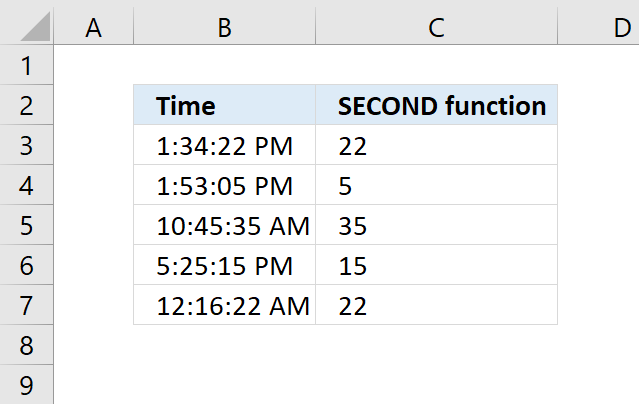Author: Oscar Cronquist Article last updated on May 04, 2018Returns an integer representing the second based on an Excel time value. The returning number is ranging from 0 to 59.

Formula in cell C3:

=SECOND(B3)

### Excel Function Syntax

SECOND(serial_number)

### Arguments

 serial_number Required. An Excel time value that you want to extract the second from.

### Comments

Excel time (serial_number) is actually a number ranging between 0 and 1 in Excel and then formatted as time.

Example, 12:00 PM is represented as 0.5 because it is half of a day, you can verify this by typing 12:00 PM in a cell and then change the cell formatting to general.

This will show the value as Excel interprets it.